### Learn Data Structures and Algorithms with Golang5 (2 reviews total)
• Constantly updated with 100+ new titles each month
• Breadth and depth in over 1,000+ technologies

Golang is one of the fastest growing programming languages in the software industry. Its speed, simplicity, and reliability make it the perfect choice for building robust applications. This brings the need to have a solid foundation in data structures and algorithms with Go so as to build scalable applications. Complete with hands-on tutorials, this book will guide you in using the best data structures and algorithms for problem solving.

The book begins with an introduction to Go data structures and algorithms. You'll learn how to store data using linked lists, arrays, stacks, and queues. Moving ahead, you'll discover how to implement sorting and searching algorithms, followed by binary search trees. This book will also help you improve the performance of your applications by stringing data types and implementing hash structures in algorithm design. Finally, you'll be able to apply traditional data structures to solve real-world problems.

By the end of the book, you'll have become adept at implementing classic data structures and algorithms in Go, propelling you to become a confident Go programmer.

Publication date:
March 2019
Publisher
Packt
Pages
336
ISBN
9781789618501

## ChapterÂ 1.Â Data Structures and Algorithms

A data structure is the organization of data to reduce the storage space used and to reduce the difficulty while performing different tasks. Data structures are used to handle and work with large amounts of data in various fields, such as database management and internet indexing services.

In this chapter, we will focus on the definition of abstract datatypes, classifying data structures into linear, nonlinear, homogeneous, heterogeneous, and dynamic types. Abstract datatypes, such asÂ Container, List, Set, Map, Graph, Stack, and Queue,Â are presented in this chapter.Â  WeÂ will also cover the performance analysis of data structures, choosing the right data structures, and structural design patterns.

The reader can start writing basic algorithms using the right data structures in Go.Â Given a problem, choosing the data structure and different algorithms will be the first step. After this, doing performance analysis is the next step. Time and space analysis for different algorithms helps compare them and helps you choose the optimal one.Â It is essential to have basic knowledge of Go to get started.Â

In this chapter, we will cover the following topics:

• Classification of data structures and structural design patterns
• Representation of algorithms
• Complexity and performance analysis
• Brute force algorithms
• Divide and conquer algorithms
• Backtracking algorithms

Â

## Technical requirements

Install Go version 1.10 fromÂ https://golang.org/doc/installÂ for your operating system.Â

The code files for this chapter can be found at the following GitHub URL:Â https://github.com/PacktPublishing/Learn-Data-Structures-and-Algorithms-with-Golang/tree/master/Chapter01.

Check the installation of Go by running the hello world programÂ at https://github.com/PacktPublishing/Learn-Data-Structures-and-Algorithms-with-Golang/tree/master/hello_world:

```//main package has examples shown
// in Hands-On Data Structures and algorithms with Go book
package main

// importing fmt package
import (
"fmt"
)
// main method
func main() {
fmt.Println("Hello World")
}```

Run the following commands:

```go build
./hello_world```

The following screenshot displays the output: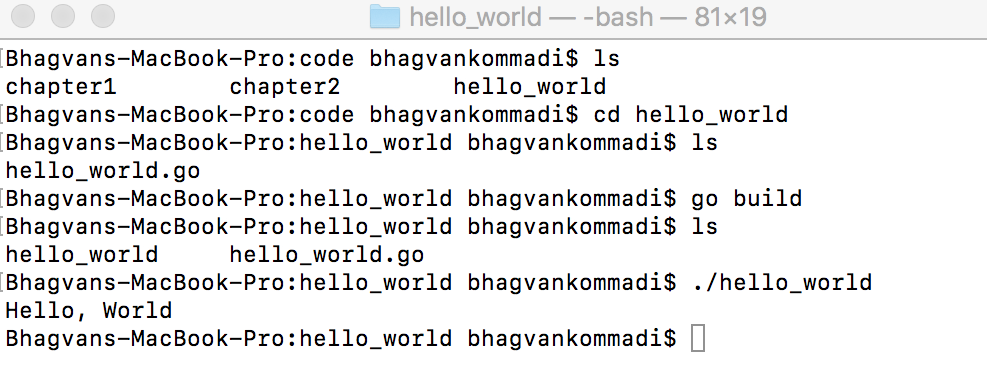Let's take a look at the classification of data structures and structural design patterns in the next section.

## Classification of data structures and structural design patterns

You can choose a data structure by using classification.Â In this section, we discuss data structure classification in detail. The design patterns related to the data structure are covered after the classification.

In the next section, we'll take a look at classification of data structures.

### Classification of data structures

The term data structure refers to the organization of data in a computer's memory, in order to retrieve it quickly for processing. It is a scheme for data organization to decouple the functional definition of a data structure from its implementation. A data structure is chosen based on the problem type and the operations performed on the data.

If the situation requires various datatypes within a data structure, we can choose heterogeneous data structures.Â Linked, ordered, and unordered lists are grouped as heterogeneous data structures.Â Linear data structures are lists, sets, tuples, queues, stacks, and heaps. Trees, tables, and containers are categorized as nonlinear data structures. Two-dimensional and multidimensional arrays are grouped as homogeneous data structures. Dynamic data structures are dictionaries, tree sets, and sequences.

The classification of Data Structures is show in the following diagram: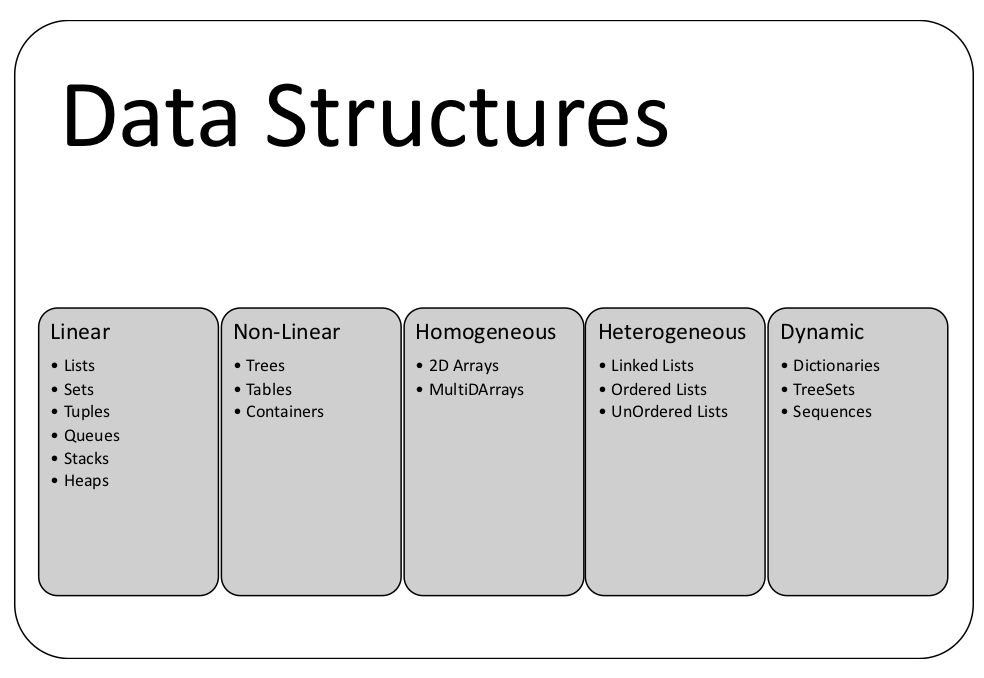Let's take a look at lists, tuples and heaps in the next sections.

#### Lists

A list is a sequence of elements. Each element can be connected to another with a link in a forward or backward direction. The element can have other payload properties. This data structure is a basic type of container. Lists have a variable length and developer can remove or add elements moreÂ easily than an array. Data items within a list need not be contiguous in memory or on disk. Linked lists were proposed byÂ Allen Newell, Cliff Shaw, and Herbert A. Simon at RAND Corporation.

Â

To get started, a list can be used in Go, as shown in the following example; elements are added through theÂ `PushBack` method on the list, which is in theÂ `container/list` package:

```//main package has examples shown
// in Hands-On Data Structures and algorithms with Go book
package main

// importing fmt and container list packages
import (
"fmt"
"container/list")

// main method
func main() {
var intList list.List
intList.PushBack(11)
intList.PushBack(23)
intList.PushBack(34)

for element := intList.Front(); element != nil; element=element.Next() {
fmt.Println(element.Value.(int))
}
}```

The list is iterated through theÂ `for` loop, and the element's value is accessed through the `Value` method.

Run the following commands:

`go run list.go`

The following screenshot displays the output: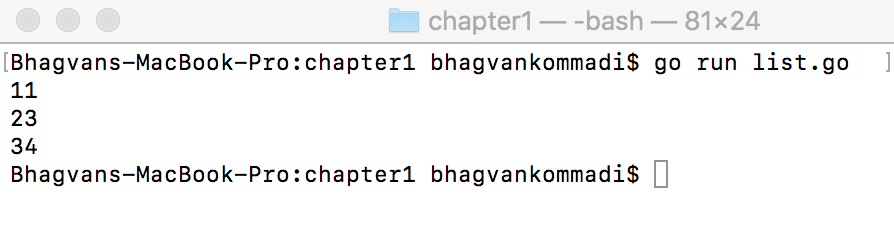Let's take a look at Tuples in the next section.

#### Tuples

A tuple is a finite sorted list of elements. ItÂ is a data structure that groups data.Â  Tuples are typically immutable sequential collections. The element has related fields of different datatypes. The only way to modify a tuple is to change the fields. Operators such as + and * can be applied to tuples. A database record is referred to as a tuple. In the following example, power series of integers are calculated and the square and cube of the integer is returned as a tuple:

```//main package has examples shown
// in Hands-On Data Structures and algorithms with Go book
package main

// importing fmt package
import (
"fmt"

)
//gets the power series of integer a and returns tuple of square of a
// and cube of a
func powerSeries(a int) (int,int) {

return a*a, a*a*a

}```

The `main` method calls the `powerSeries` method with `3` as a parameter.Â The`square`and `cube`Â values are returned from the method:

```// main method
func main() {

var square int
var cube int
square, cube = powerSeries(3)

fmt.Println("Square ", square, "Cube", cube)

}```

Â

Â

Â

Â

Run the following commands:

`go run tuples.go`

The following screenshot displays the output: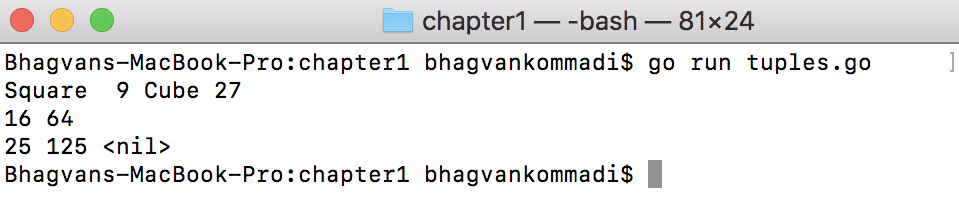The tuples can be named in the `powerSeries`function, as shown in the following code:

```func powerSeries(a int) (square int, cube int) {

square = a*a

cube = square*a

return

}```

If there is an error, it can be passed with tuples, as shown in the following code:

```func powerSeries(a int) (int, int, error) {

square = a*a

cube = square*a

return square,cube,nil

}```

#### Heaps

A heap is a data structure that is based on the `heap` property. The heap data structure is used in selection, graph, and k-way merge algorithms. Operations such as finding, merging, insertion, key changes, and deleting are performed on heaps. Heaps are part of theÂ `container/heap`Â package in Go. According to the heap order (maximum heap) property, the value stored at each node is greater than or equal to its children.

If the order is descending, it is referred to as a maximum heap; otherwise, it's a minimum heap.Â The heap data structure was proposed by J.W.J. Williams in 1964 for a heap sorting algorithm. It is not a sorted data structure, but partially ordered. The following example shows how to use the `container/heap` package to create a heap data structure:

```//main package has examples shown
//in Hands-On Data Structures and algorithms with Go book
package main

// importing fmt package and container/heap
import (
"container/heap"
"fmt"
)
// integerHeap a type
type IntegerHeap []int

// IntegerHeap method - gets the length of integerHeap
func (iheap IntegerHeap) Len() int { return len(iheap) }

// IntegerHeap method - checks if element of i index is less than j index
func (iheap IntegerHeap) Less(i, j int) bool { return iheap[i] < iheap[j] }
// IntegerHeap method -swaps the element of i to j index
func (iheap IntegerHeap) Swap(i, j int) { iheap[i], iheap[j] = iheap[j], iheap[i] }```

`IntegerHeap` has a `Push` method that pushes the item with the interface:

```//IntegerHeap method -pushes the item
func (iheap *IntegerHeap) Push(heapintf interface{}) {

*iheap = append(*iheap, heapintf.(int))
}
//IntegerHeap method -pops the item from the heap
func (iheap *IntegerHeap) Pop() interface{} {
var n int
var x1 int
var previous IntegerHeap = *iheap
n = len(previous)
x1 = previous[n-1]
*iheap = previous[0 : n-1]
return x1
}

// main method
func main() {
var intHeap *IntegerHeap = &IntegerHeap{1,4,5}

heap.Init(intHeap)
heap.Push(intHeap, 2)
fmt.Printf("minimum: %d\n", (*intHeap))
for intHeap.Len() > 0 {
fmt.Printf("%d \n", heap.Pop(intHeap))
}
}```

Run the following commands:

`go run heap.go`

The following screenshot displays the output: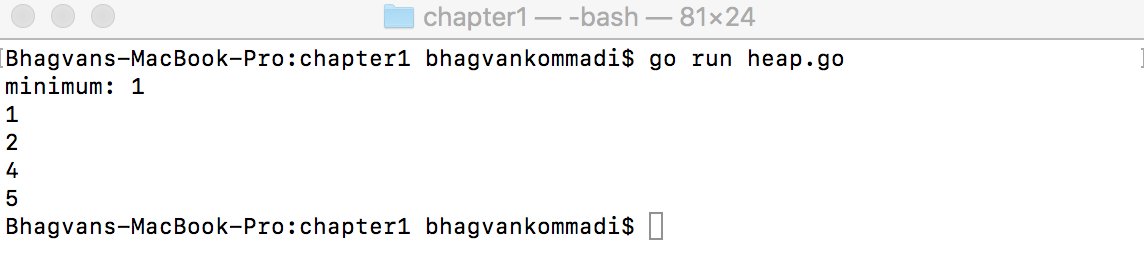Let's take a look at structural design patterns in the next section

### Structural design patterns

Structural design patterns describe the relationships between the entities. They are used to form large structures using classes and objects. These patterns are used to create a system with different system blocks in a flexible manner.Â Adapter, bridge, composite, decorator, facade, flyweight, private class data, and proxy are the Gang of Four (GoF) structural design patterns.Â The private class data design pattern is the other design pattern covered in this section.

We will take a look at adapter and bridge design patterns in the next sections.

Â

The adapter pattern provides a wrapper with an interface required by the API client to link incompatible types and act as a translator between the two types. The adapter uses the interface of a class to be a class with another compatible interface. When requirements change, there are scenarios where class functionality needs to be changed because of incompatible interfaces.

The dependency inversion principle can be adhered to by using the adapter pattern, when a class defines its own interface to the next level module interface implemented by an `adapter` class. Delegation is the other principle used by the adapter pattern. Multiple formats handling source-to-destination transformations are the scenarios where the adapter pattern is applied.

• Target is the interface that the client calls and invokes methods on the adapter and adaptee.
• The client wants the incompatible interface implemented by the adapter.
• The adapter translates the incompatible interface of the adaptee into an interface that the client wants.

Let's say you have anÂ `IProcessor`Â interface with a `process`method, theÂ `Adapter`Â class implements the `process`Â method and has anÂ `Adaptee`Â instance as an attribute. The `Adaptee` class has a `convert`Â method and anÂ `adapterType`Â instance variable. The developer while using the API client calls the `process`Â interface methodÂ to invoke `convert` on `Adaptee`. The code is as follows:

```//main package has examples shown
// in Hands-On Data Structures and algorithms with Go book
package main
// importing fmt package
import (
"fmt"
)
//IProcess interface
type IProcess interface {
process()
}
}```

Â

Â

Â

Â

Â

Â

The `Adapter` class has aÂ `process`Â method that invokes theÂ `convert` method on `adaptee`:

```//Adapter class method process
}
}
}
// main method
func main() {
processor.process()
}```

Run the following commands:

`go run adapter.go`

The following screenshot displays the output: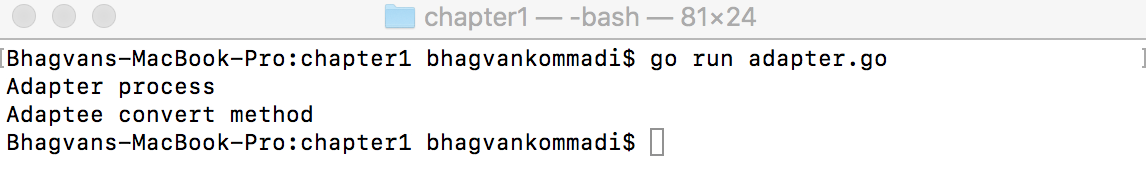Let's take a look at Bridge pattern in the next section.

#### Bridge

Bridge decouples the implementation from the abstraction. The abstract base class can be subclassed to provide different implementations and allow implementation details to be modified easily. The interface, which is a bridge, helps in making the functionality of concrete classes independent from the interface implementer classes. The bridge patterns allow the implementation details to change at runtime.

Â

The bridge pattern demonstrates the principle, preferring composition over inheritance. It helps in situations where one should subclass multiple times orthogonal to each other. Runtime binding of the application, mapping of orthogonal class hierarchies, and platform independence implementation are the scenarios where the bridge pattern can be applied.

The bridge pattern components are abstraction, refined abstraction, implementer, and concrete implementer. Abstraction is the interface implemented as an abstract class that clients invoke withÂ the method on the concrete implementer. Abstraction maintains a has-aÂ relationship with the implementation, instead of anÂ is-aÂ relationship. The has-aÂ relationship is maintained by composition. Abstraction has a reference of the implementation. Refined abstraction provides more variations than abstraction.

Let's say`IDrawShape` is an interface with theÂ `drawShape`Â method.Â Â `DrawShape` implements the `IDrawShape` interface. We create anÂ `IContour`bridge interfaceÂ with theÂ `drawContour`Â method. The contour class implements theÂ `IContour` interface. The `ellipse` class will have a, b , r properties and `drawShape` (an instance of `DrawShape`). The `ellipse` class implements theÂ `contour`Â bridge interface to implement theÂ `drawContour`Â method. TheÂ Â `drawContour`Â method calls theÂ `drawShape`Â method on theÂ `drawShape`Â instance.

The following code demonstrates the bridge implementation:Â

```//main package has examples shown
// in Hands-On Data Structures and algorithms with Go book
package main
// importing fmt package
import (
"fmt"
)
//IDrawShape interface
type IDrawShape interface {
drawShape(x float32,y float32)
}
//DrawShape struct
type DrawShape struct{}```
##### drawShape method

The `drawShape` method draws the shape given the coordinates, as shown in the following code:

```// DrawShape struct has method draw Shape with float x and y coordinates
func (drawShape DrawShape) drawShape(x float32, y float32) {
fmt.Println("Drawing Shape")
}
//IContour interace
type IContour interface {
drawContour(x float32 ,y float32)
resizeByFactor(factor int)
}
//DrawContour struct
type DrawContour struct {
x float32
y float32
shape DrawShape
factor int
}```
##### drawContour method

TheÂ `drawContour` methodÂ of theÂ `DrawContour`Â classcalls theÂ `drawShape`Â method on the `shape` instance, this is shown in the following code:

```//DrawContour method drawContour given the coordinates
func (contour DrawContour) drawContour(x float32,y float32) {
fmt.Println("Drawing Contour")
contour.shape.drawShape(contour.x,contour.y)
}
//DrawContour method resizeByFactor given factor
func (contour DrawContour) resizeByFactor(factor int) {
contour.factor = factor
}
// main method
func main() {
var x = float32{1,2,3,4,5}
var y = float32{1,2,3,4,5}
var contour IContour = DrawContour{x,y,DrawShape{},2}
contour.drawContour(x,y)
contour.resizeByFactor(2)
}```

Run the following commands:

`go run bridge.go`

Â

The following screenshot displays the output: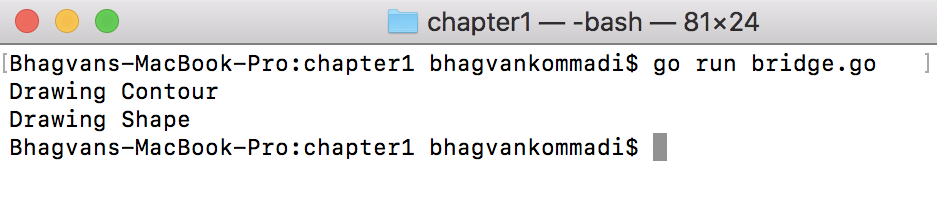We will take a look at Composite, Decorator, Facade and Flyweight design patterns in the next sections.

#### Composite

A composite is a group of similar objects in a single object. Objects are stored in a tree form to persist the whole hierarchy. The composite pattern is used to change a hierarchical collection of objects. TheÂ composite pattern is modeled on a heterogeneous collection. New types of objects can be added without changing the interface and the client code. You can use the composite pattern, for example, for UI layouts on the web, for directory trees, and for managing employees across departments. The pattern provides a mechanism to access the individual objects and groups in a similar manner.

The composite pattern comprises the `component` interface, `component` class, composite, and client:

• The `component` interface defines the default behavior of all objects and behaviors for accessing the components of the composite.
• The `composite` and `component` classes implement the `component` interface.
• The client interacts with the component interface to invoke methods in the composite.

Let's say there is anÂ `IComposite`Â interface with the `perform` method and `BranchClass`Â that implements `IComposite`Â  and has theÂ `addLeaf`, `addBranch`, and `perform`Â methods. The `Leaflet` class implements `IComposite` with the `perform` method.Â `BranchClass` has a one-to-many relationship with `leafs` and `branches`. Iterating over the branch recursively, one can traverse the composite tree, as shown in the following code:

```//main package has examples shown
// in Hands-On Data Structures and algorithms with Go book
package main
// importing fmt package
import (
"fmt"
)
// IComposite interface
type IComposite interface {
perform()
}
// Leaflet struct
type Leaflet struct {
name string
}
// Leaflet class method perform
func (leaf *Leaflet) perform() {
fmt.Println("Leaflet " + leaf.name)
}
// Branch struct
type Branch struct {
leafs []Leaflet
name string
branches[]Branch
}```

TheÂ `perform` method of theÂ `Branch`Â classÂ calls theÂ `perform`Â method on `branch` and `leafs`, as seen in the code:

```// Branch class method perform
func (branch *Branch) perform() {
fmt.Println("Branch: " + branch.name)
for _, leaf := range branch.leafs {
leaf.perform()
}
for _, branch := range branch.branches {
branch.perform()
}
}
// Branch class method add leaflet
func (branch *Branch) add(leaf Leaflet) {
branch.leafs = append(branch.leafs, leaf)
}```

As shown in the following code, theÂ `addBranch` methodÂ of theÂ `Branch`Â classÂ adds a new `branch`:

```//Branch class method addBranch branch
func (branch *Branch) addBranch(newBranch Branch) {
branch.branches = append(branch.branches,newBranch)
}
//Branch class method getLeaflets
func (branch *Branch) getLeaflets() []Leaflet {
return branch.leafs
}
// main method
func main() {
var branch = &Branch{name:"branch 1"}
var leaf1 = Leaflet{name:"leaf 1"}
var leaf2 = Leaflet{name:"leaf 2"}
var branch2 = Branch{name:"branch 2"}
branch.perform()
}```

Run the following commands:

`go run composite.go`

The following screenshot displays the output: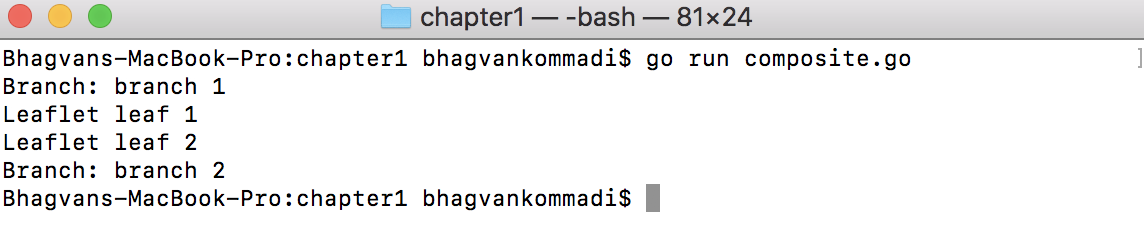Let's take a look at Decorator pattern in the next section.

#### Decorator

In a scenario where class responsibilities are removed or added, the decorator pattern is applied. The decorator pattern helps with subclassing whenÂ modifying functionality, instead of static inheritance. An object can have multiple decorators and run-time decorators. The single responsibility principle can be achieved using a decorator.Â TheÂ decorator can be applied toÂ window components and graphical object modeling. The decorator pattern helps with modifying existing instance attributes and adding new methods at run-time.

Â

The decorator pattern participants are the component interface, the concrete component class, and theÂ `decorator` class:

• The concrete component implements the component interface.
• The `decorator` class implements the component interface and provides additional functionality in the same method or additional methods. The decorator base can be a participant representing the base class for all decorators.

Let 's say`IProcess` is an interface with the `process` method. `ProcessClass` implements an interface with the `process` method. `ProcessDecorator` implements the process interface and has an instance of `ProcessClass`. `ProcessDecorator` can add more functionality thanÂ `ProcessClass`, as shown in the following code:

```//main package has examples shown
// in Hands-On Data Structures and algorithms with Go book
package main
// importing fmt package
import (
"fmt"
)
// IProcess Interface
type IProcess interface {
process()
}
//ProcessClass struct
type ProcessClass struct{}
//ProcessClass method process
func (process *ProcessClass) process() {
fmt.Println("ProcessClass process")
}
//ProcessDecorator struct
type ProcessDecorator struct {
processInstance *ProcessClass
}```

In the following code, the `ProcessDecorator` class `process`methodÂ invokes the `process` method on the decorator instance of `ProcessClass`:

``` //ProcessDecorator class method process
func (decorator *ProcessDecorator) process() {
if decorator.processInstance == nil {
fmt.Println("ProcessDecorator process")
} else {
fmt.Printf("ProcessDecorator process and ")
decorator.processInstance.process()
}
}
//main method
func main() {
var process = &ProcessClass{}
var decorator = &ProcessDecorator{}
decorator.process()
decorator.processInstance = process
decorator.process()
}```

Run the following commands:

`go run decorator.go`

The following screenshot displays the output: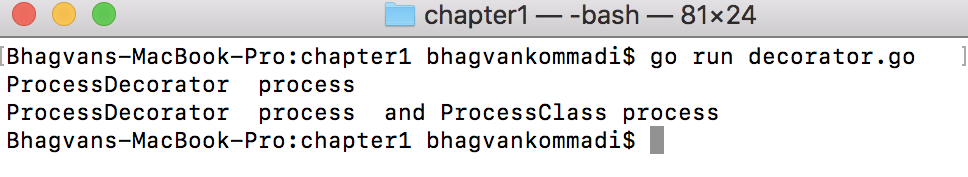Let's take a look at Facade pattern in the next section.

Facade is used to abstract subsystem interfaces with a helper. The facade design pattern is used in scenarios when the number of interfaces increases and the system gets complicated. Facade is an entry point to different subsystems, and it simplifies the dependencies between the systems. The facade pattern provides an interface that hides the implementation details of the hidden code.

A loosely coupled principle can be realized with a facade pattern. You can use a facade to improve poorly designed APIs. In SOA, a service facade can be used to incorporate changes to the contract and implementation.

Â

TheÂ facade pattern is made up of theÂ `facade` class, module classes, and a client:

• The facade delegates the requests from the client to the module classes. The `facade` class hides the complexities of the subsystem logic and rules.
• Module classes implement the behaviors and functionalities of the module subsystem.
• The client invokes the `facade` method. The `facade` class functionality can be spread across multiple packages and assemblies.

For example, account, customer, and transaction are the classes that have account, customer, and transaction creation methods. `BranchManagerFacade` can be used by the client to create an account, customer, and transaction:

```//main package has examples shown
// in Hands-On Data Structures and algorithms with Go book
package main
// importing fmt package
import (
"fmt"
)
//Account struct
type Account struct{
id string
accountType string
}
//Account class method create - creates account given AccountType
func (account *Account) create(accountType string) *Account{
fmt.Println("account creation with type")
account.accountType = accountType
return account
}
//Account class method getById given id string
func (account *Account) getById(id string) *Account {
fmt.Println("getting account by Id")
return account
}```

The `account` class has theÂ `deleteById`Â method, which is used to delete an account with a given ID, as shown in the following code:

``` //Account class method deleteById given id string
func (account *Account) deleteById(id string)() {
fmt.Println("delete account by id")
}
//Customer struct
type Customer struct{
name string
id int
}```

In the following code, the `customer` class has a method that creates a new customer with `name`:

```//Customer class method create - create Customer given name
func (customer *Customer) create(name string) *Customer {
fmt.Println("creating customer")
customer.name = name
return customer
}
//Transaction struct
type Transaction struct{
id string
amount float32
srcAccountId string
destAccountId string
}```

As shown in the following code, the `transaction` class has theÂ `create`Â method for creating a transaction:

```//Transaction class method create Transaction
func (transaction *Transaction) create(srcAccountId string, destAccountId string,amount float32) *Transaction {
fmt.Println("creating transaction")
transaction.srcAccountId = srcAccountId
transaction.destAccountId = destAccountId
transaction.amount = amount
return transaction
}
account *Account
customer *Customer
transaction *Transaction
}
}```

Â

Â

Â

Â

`BranchManagerFacade` has theÂ `createCustomerAccount`Â method, which calls theÂ `create` method on theÂ `customer` class instance, as shown in the following code:

```//BranchManagerFacade class method createCustomerAccount
return customer, account
}
return transaction
}```

The `main` method calls the `NewBranchManagerFacade` method to create a facade. The methods on `facade` are invoked to create `customer` and `account`:

```//main method
func main() {
var customer *Customer
var account *Account
"Savings")
fmt.Println(customer.name)
fmt.Println(account.accountType)
fmt.Println(transaction.amount)
}```

Run the following commands:

`go run facade.go`

The following screenshot displays the output: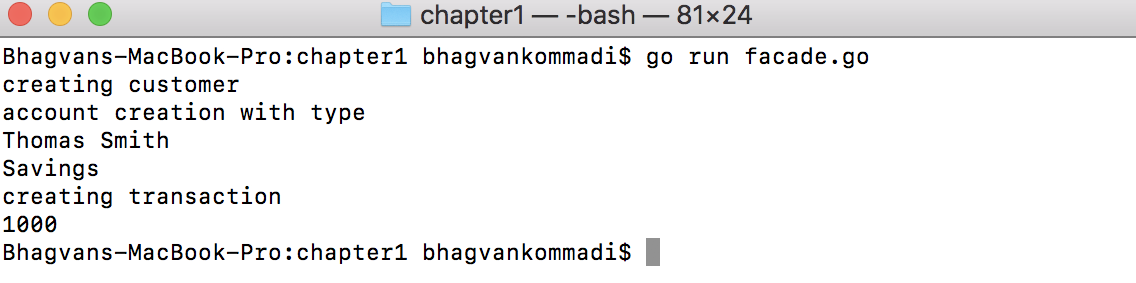Â

Â

Let's take a look at Flyweight pattern in the next section.

#### Flyweight

Flyweight is used to manage the state of an object with high variation. The pattern allows us to share common parts of the object state among multiple objects, instead of each object storing it. Variable object data is referred to as extrinsic state, and the rest of the object state is intrinsic. Extrinsic data is passed to flyweight methods and will never be stored within it. Flyweight pattern helps reduce the overall memory usage and the object initializing overhead. The pattern helps create interclass relationships and lower memory to a manageable level.

Flyweight objects are immutable. Value objects are a good example of the flyweight pattern. Flyweight objects can be created in a single thread mode, ensuring one instance per value. In a concurrent thread scenario, multiple instances are created. This is based on the equality criterion of flyweight objects.

The participants of the flyweight pattern are the `FlyWeight` interface, `ConcreteFlyWeight`, `FlyWeightFactory`, and theÂ `Client` classes:

• The `FlyWeight` interface has a method through which flyweights can get and act on the extrinsic state.
• `ConcreteFlyWeight` implements the `FlyWeight` interface to represent flyweight objects.
• `FlyweightFactory` is used to create and manage flyweight objects. The client invokes `FlyweightFactory` to get a flyweight object. `UnsharedFlyWeight` can have a functionality that is not shared.
• `Client`classes

Let's say`DataTransferObject` is an interface with theÂ `getId` method. `DataTransferObjectFactory` creates a data transfer object through `getDataTransferObject` by the `DTO` type. The `DTO` types are customer, employee, manager, and address, as shown in the following code:

```//main package has examples shown
// in Hands-On Data Structures and algorithms with Go book
package main
// importing fmt package
import (
"fmt"
)
//DataTransferObjectFactory struct
type DataTransferObjectFactory struct {
pool map[string] DataTransferObject
}
//DataTransferObjectFactory class method getDataTransferObject
func (factory DataTransferObjectFactory) getDataTransferObject(dtoType string) DataTransferObject {
var dto = factory.pool[dtoType]
if dto == nil {
fmt.Println("new DTO of dtoType: " + dtoType)
switch dtoType{
case "customer":
factory.pool[dtoType] = Customer{id:"1"}
case "employee":
factory.pool[dtoType] = Employee{id:"2"}
case "manager":
factory.pool[dtoType] = Manager{id:"3"}
}
dto = factory.pool[dtoType]
}

return dto
}```

In the following code, the `DataTransferObject` interface is implemented by the `Customer` class:

```// DataTransferObject interface
type DataTransferObject interface {
getId() string
}
//Customer struct
type Customer struct {
id string //sequence generator
name string
ssn string
}
// Customer class method getId
func (customer Customer) getId() string {
//fmt.Println("getting customer Id")
return customer.id
}
//Employee struct
type Employee struct {
id string
name string
}
//Employee class method getId
func (employee Employee) getId() string {
return employee.id
}
//Manager struct
type Manager struct {
id string
name string
dept string
}```

The `DataTransferObject` interface is implemented by theÂ `Manager` class, as shown in the following code:

```//Manager class method getId
func (manager Manager) getId() string {
return manager.id
}
id string
streetLine1 string
streetLine2 string
state string
city string
}
}
//main method
func main() {
var factory = DataTransferObjectFactory{make(map[string]DataTransferObject)}
var customer DataTransferObject = factory.getDataTransferObject("customer")
fmt.Println("Customer ",customer.getId())
var employee DataTransferObject = factory.getDataTransferObject("employee")
fmt.Println("Employee ",employee.getId())
var manager DataTransferObject = factory.getDataTransferObject("manager")
fmt.Println("Manager",manager.getId())
}```

Â

Â

Â

Run the following commands:

`go run flyweight.go`

The following screenshot displays the output: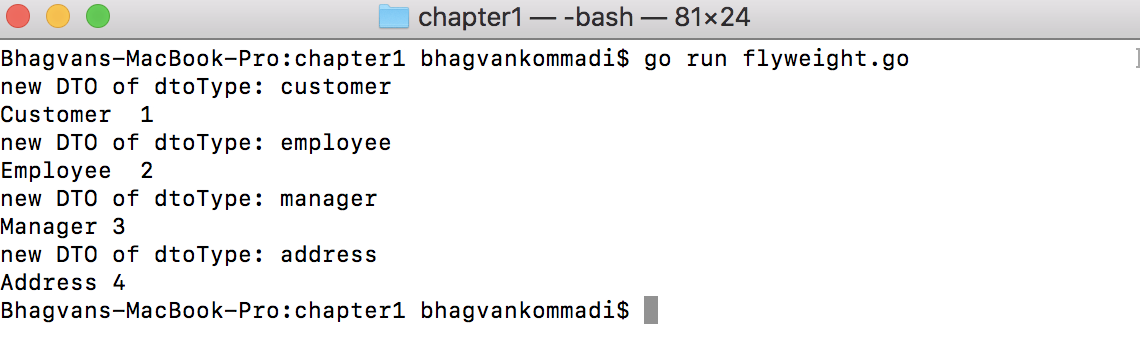We will take a look at Private class and Proxy data patterns in the next sections.

#### Private class data

The private class data pattern secures the data within a class. This pattern encapsulates the initialization of the class data. The write privileges of properties within the private class are protected, and properties are set during construction. The private class pattern prints the exposure of information by securing it in a class that retains the state. The encapsulation of class data initialization is a scenario where this pattern is applicable.

`Account` is a class with account details and a customer name. `AccountDetails` is the private attribute of `Account`Â , and `CustomerName` is the public attribute. JSON marshaling of `Account` hasÂ `CustomerName` as a public property. `AccountDetails` is the package property in Go (modeled as private class data):

```//main package has examples shown
// in Hands-On Data Structures and algorithms with Go book
package main
// importing fmt and encoding/json packages
import (
"encoding/json"
"fmt"
)
//AccountDetails struct
type AccountDetails struct {
id string
accountType string
}
//Account struct
type Account struct {
details *AccountDetails
CustomerName string
}
// Account class method setDetails
func (account *Account) setDetails(id string, accountType string) {
account.details = &AccountDetails{id, accountType}
}```

As shown in the following code, the `Account` class has theÂ `getId`Â method, which returns theÂ `id`Â private class attribute:

```//Account class method getId
func (account *Account) getId() string{
return account.details.id
}
//Account class method getAccountType
func (account *Account) getAccountType() string{
return account.details.accountType
}```

The `main` method calls theÂ `Account` initializer with `CustomerName`. The details of the account are set details with theÂ `setDetails` method:

```// main method
func main() {
var account *Account = &Account{CustomerName: "John Smith"}
account.setDetails("4532","current")
jsonAccount, _ := json.Marshal(account)
fmt.Println("Private Class hidden",string(jsonAccount))
fmt.Println("Account Id",account.getId())
fmt.Println("Account Type",account.getAccountType())
}```

Run the following commands:

`go run privateclass.go`

The following screenshot displays the output: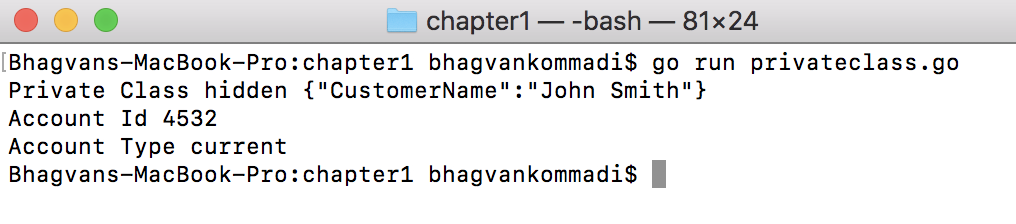Let's take a look at Proxy pattern in the next section.

#### Proxy

The proxy pattern forwards to a real object and acts as an interface to others. The proxy pattern controls access to an object and provides additional functionality. The additional functionality can be related to authentication, authorization, and providing rights of access to the resource-sensitive object. The real object need not be modified while providing additional logic. Remote, smart, virtual, and protection proxies are the scenarios where this pattern is applied. It is also used to provide an alternative to extend functionality with inheritance and object composition. A proxy object is also referred to as a surrogate, handle, or wrapper.

The proxy pattern comprises theÂ subject interface, theÂ `RealSubject` class, and theÂ `Proxy` class:

• Subject is an interface for theÂ `RealObject` and `Proxy` class.
• The `RealSubject` object is created and maintained as a reference in theÂ `Proxy` class.Â `RealSubject` is resource sensitive, required to be protected, and expensive to create.Â Â `RealObject` is a class that implements theÂ `IRealObject` interface. It has a `performAction` method.Â
• `VirtualProxy` is used to access `RealObject` and invoke theÂ `performAction` method.

The following code shows an implementation of proxy pattern:

``` //main package has examples shown
// in Hands-On Data Structures and algorithms with Go book
package main
// importing fmt package
import (
"fmt"
)
//IRealObject interface
type IRealObject interface {
performAction()
}
//RealObject struct
type RealObject struct{}
RealObject class implements IRealObject interface. The class has method performAction.
//RealObject class method performAction
func (realObject *RealObject) performAction() {
fmt.Println("RealObject performAction()")
}
//VirtualProxy struct
type VirtualProxy struct {
realObject *RealObject
}
//VirtualProxy class method performAction
func (virtualProxy *VirtualProxy) performAction() {
if virtualProxy.realObject == nil {
virtualProxy.realObject = &RealObject{}
}
fmt.Println("Virtual Proxy performAction()")
virtualProxy.realObject.performAction()
}
// main method
func main() {
var object VirtualProxy = VirtualProxy{}
object.performAction()
}```

Run the following commands:

`go run virtualproxy.go`

The following screenshot displays the output: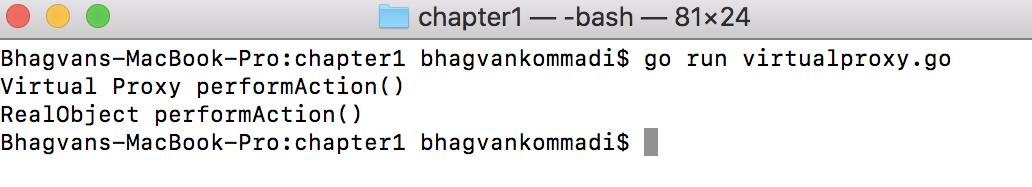Now that we know the classification of data structures and the design patterns used, let's go ahead and take a look at the representation of algorithms.Â

## Representation of algorithms

A flow chart and pseudo code are methods of representing algorithms.Â An algorithm shows the logic of how a problem is solved. A flow chart has different representation symbols such as Entry, Exit, Task, Input/Output, Decision Point, and Inter Block. A structured program consists of a series of these symbols to perform a specific task. Pseudo code has documentation, action, and flow control keywords to visualize an algorithm. The documentation keywords are TASK and REM. SET, PUT, and GET are the action keywords.

Let's take a look at the different representations of algorithms, that is, flow charts and Pseudo code in the next sections.

### Flow chart

The flow control keywords areSET, LOOP, (WHILE, UNTIL), REP, and POST.Â The following flow chart shows a formula or an algorithm to calculate the dividend given a number of shares, the face value, and the dividend percentage. The start and end are the Entry and Exit symbols. The input number of shares, share face value, and dividend percentage use the Input symbol. The compute dividend and output dividend use the Task symbol and Output symbol respectively: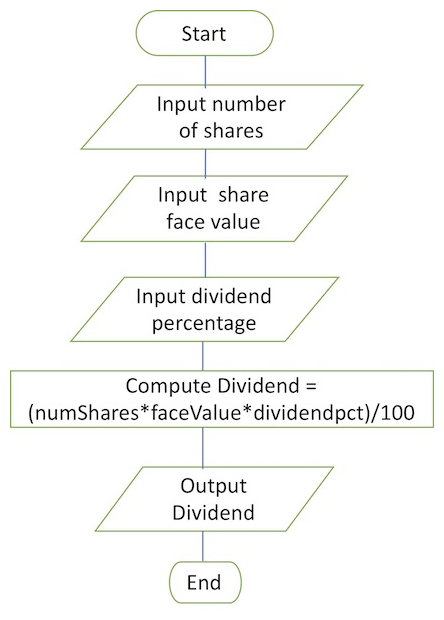Â

Â In the next section, we'llÂ take a look at pseudo code, representation of algorithms.

### Pseudo code

Pseudo code is a high-level design of a program or algorithm. Sequence and selection are two constructs used in pseudo code. Pseudo code is easier than a flow chart visualizes the algorithm while pseudo code can be easily modified and updated. Errors in design can be caught very early in pseudo code. This saves the cost of fixing defects later.

To give an example, we want to find the `max` value in an array of length n. The pseudo code will be written as follows:

```maximum(arr) {
n <- len(arr)
max <- arr
for k <- 0,n do{
Ifarr[k] > max {
max <- arr[k]
}
}
return max
}```

Now that we know the different ways to represent the algorithm, let's take a look at how we can monitor its complexity and performance in the next section.

## Complexity and performance analysis

The efficiency of an algorithm is measured through various parameters, such as CPU time, memory, disk, and network. The complexity is how the algorithm scales when the number of input parameters increases. Performance is a measure of time, space, memory, and other parameters. Algorithms are compared by their processing time and resource consumption. Complexity measures the parameters and is represented by the Big O notation.

### Complexity analysis of algorithms

The complexity of an algorithm is measured by the speed of the algorithm. Typically, the algorithm will perform differently based on processor speed, disk speed, memory, and other hardware parameters. Hence, asymptotical complexity is used to measure the complexity of an algorithm. An algorithm is a set of steps to be processed by different operations to achieve a task. The time taken for an algorithm to complete is based on the number of steps taken.

Let's say an algorithm iterates through an array,Â `m`, of size `10`and update the elements to the sum of index and`200`. The computational time will be 10*t, where tÂ is the time taken to addÂ two integers and update them to an array. The next step will be printing them after iterating over an array. TheÂ tÂ  time parameter will vary with the hardware of the computer used. Asymptotically, the computational time grows as a factor of `10`, as shown in the following code:

```//main package has examples shown
// in Hands-On Data Structures and algorithms with Go book
package main
// importing fmt package
import (
"fmt"
)
// main method
func main() {
var m int
var k int
for k = 0; k < 10; k++ {
m[k] = k + 200
fmt.Printf("Element[%d] = %d\n", k, m[k] )
}
}```

Run the following commands:

`go run complexity.go`

The following screenshot displays the output: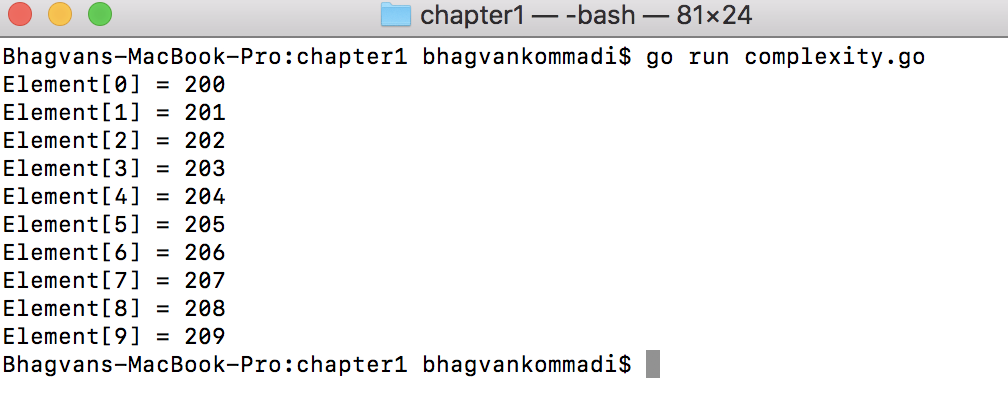Let's take a look at the different complexity types in the next sections.

#### Big O notation

TheÂ T(n) time function represents the algorithm complexity based on Big O notation. T(n) = O(n) states that an algorithm has a linear time complexity. Using Big O notation, the constant time, linear time, logarithmic time, cubic time, and quadratic time complexity are different complexity types for an algorithm.

Linear time,Â O(n), is used as a measure of complexity in scenarios such as linear search, traversing, and finding the minimum and maximum number of array elements. ArrayList and queue are data structures that have these methods. An algorithm that has logarithmic time,Â O(log n), is a binary search in a tree data structure. Bubble sort, selection sort, and insertion sort algorithms have complexity of quadratic time,Â O(n2). Big Omega Î© and big Theta Î˜ are notations for the lower and upper bounds for a particular algorithm.

The worst case, best case, average case, and amortized run-time complexity is used for analysis of algorithms. Amortized run-time complexity is referred to as 2n. Asymptotically, it will tend to O(1).

Big O notation is also used to determine how much space is consumed by the algorithm. This helps us find the best and worst case scenarios, relative to space and time.

Let's take a look at linear complexity in the next section.

Â

#### Linear complexity

An algorithm is of linear complexity if the processing time or storage space is directly proportional to the number of input elements to be processed. In Big O notation, linear complexity is presented as O(n). String matching algorithms such as the Boyer-Moore and Ukkonen have linear complexity.

Linear complexity,Â O(n), is demonstrated in an algorithm as follows:

```//main package has examples shown
// in Go Data Structures and algorithms book
package main
// importing fmt package
import (
"fmt"
)
// main method
func main() {
var m int
var k int
for k = 0; k < 10; k++ {
m[k] = k * 200
fmt.Printf("Element[%d] = %d\n", k, m[k] )
}
}```

Run the following commands:

`go run linear_complexity.go`

The following screenshot displays the output: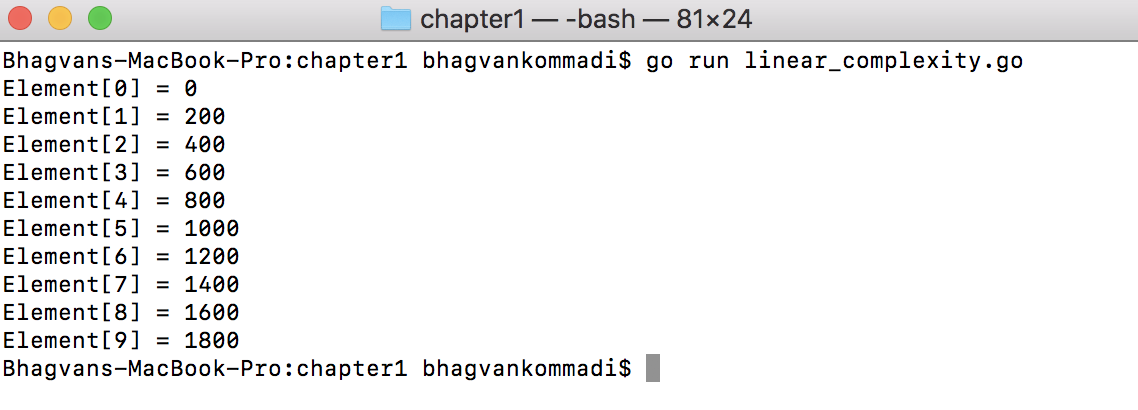Â

Let's take a look at quadratic complexity in the next section.

An algorithm is of quadratic complexity if the processing time is proportional to the square of the number of input elements.Â In the following case, the complexity of the algorithm is 10*10 = 100. The two loops have a maximum ofÂ `10`. The quadratic complexity for a multiplication table of n elements is O(n2).

Quadratic complexity,Â O(n2), is shown in the following example:

```//main package has examples shown
// in Go Data Structures and algorithms book
package main
// importing fmt package
import (
"fmt"
)
// main method
func main() {
var k,l int
for k = 1; k <= 10; k++ {
fmt.Println(" Multiplication Table", k)
for l=1; l <= 10; l++ {
var x int = l *k
fmt.Println(x)
}
}
}```

Run the following commands:

`go run quadratic_complexity.go`

The following screenshot displays the output: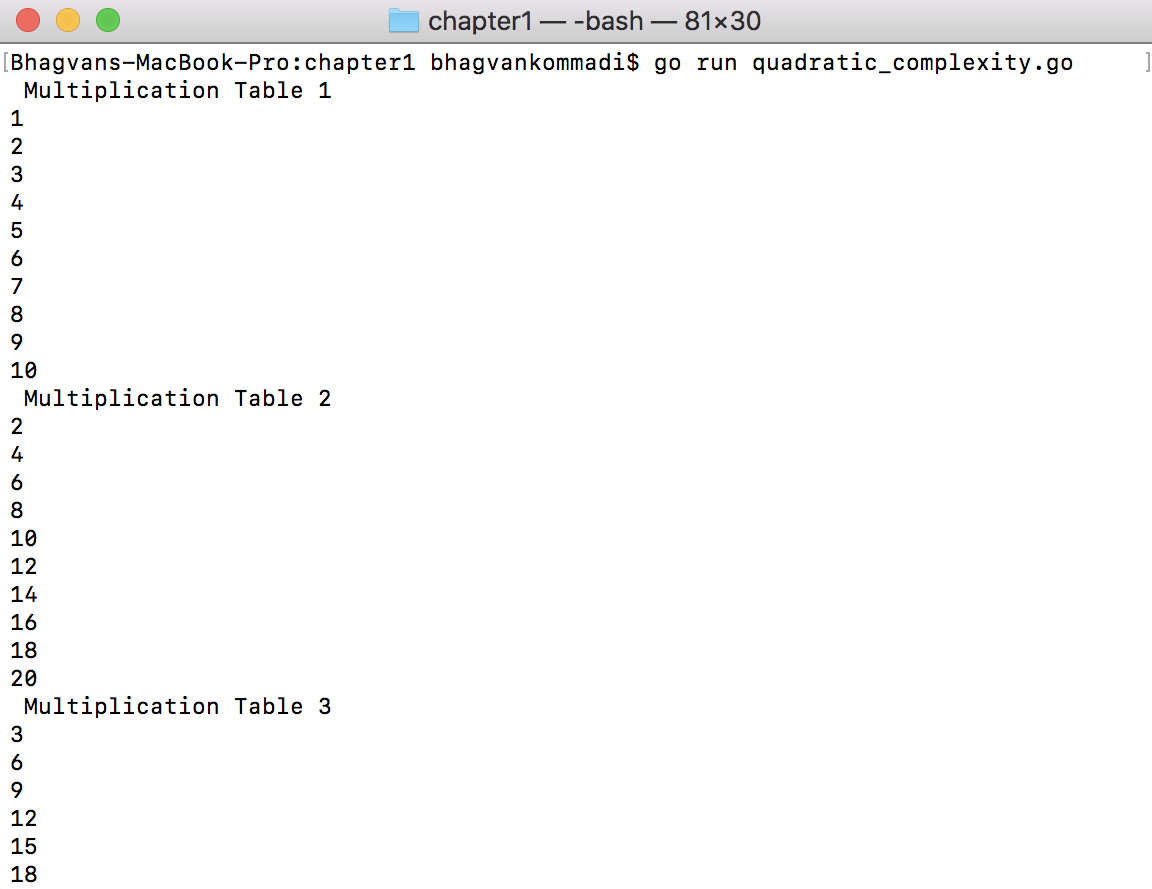Let's take a look at cubic complexity in the next section.

#### Cubic complexity

In the case of cubic complexity, the processing time of an algorithm is proportional to the cube of the input elements.Â The complexity of the following algorithm is 10*10*10 = 1,000. The three loops have a maximum of 10. The cubic complexity for a matrix update is O(n3).

Cubic complexity O(n3) is explained in the following example:

```//main package has examples shown
// in Hands-On Data Structures and algorithms with Go book
package main
// importing fmt package
import (
"fmt"
)
// main method
func main() {
var k,l,m int
var arr int
for k = 0; k < 10; k++ {
for l=0; l < 10; l++ {
for m=0; m < 10; m++ {
arr[k][l][m] = 1
fmt.Println("Element value ",k,l,m," is", arr[k][l][m])
}
}
}
}```

Run the following commands:

`go run cubic_complexity.go`

The following screenshot displays the output: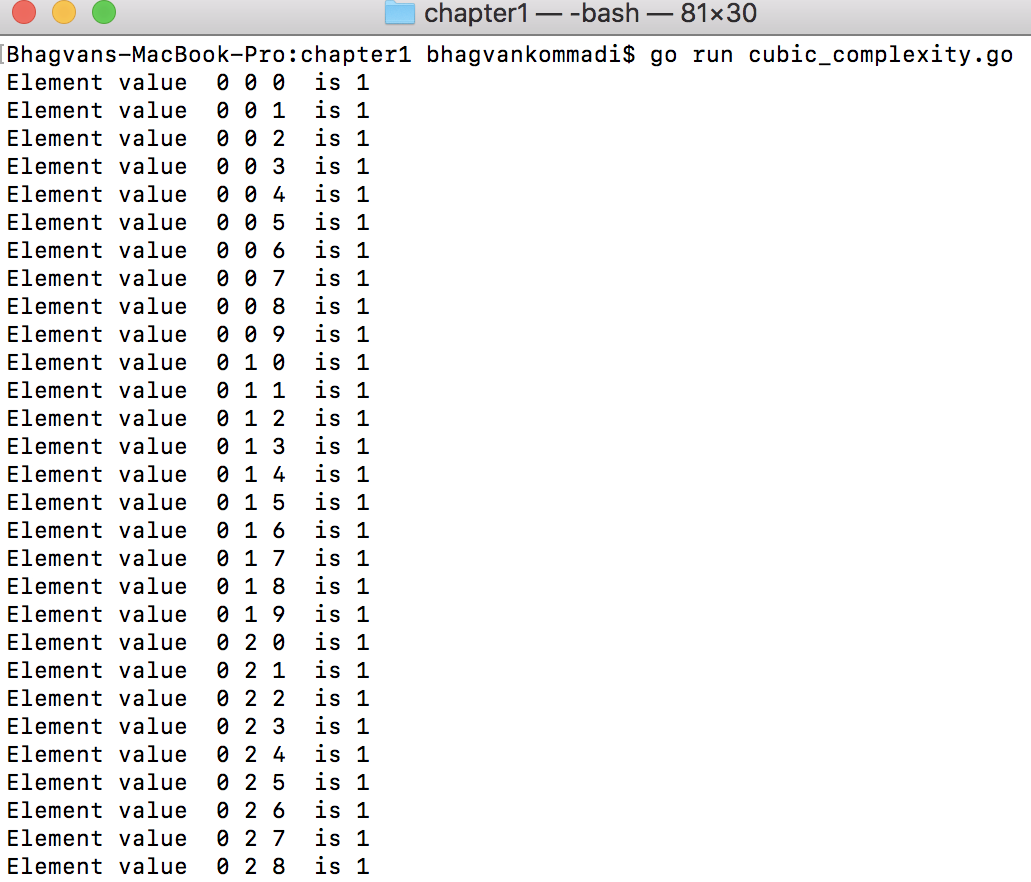Let's take a look at logarithmic complexity in the next section.

#### Logarithmic complexity

An algorithm is of logarithmic complexity if the processing time is proportional to the logarithm of the input elements. The logarithm base is typically 2. The following tree is a binary tree with `LeftNode`and`RightNode`. The insert operation is of O(log n) complexity, where n is the number of nodes.

Logarithmic complexity is presented as follows:

```//main package has examples shown
// in Hands-On Data Structures and algorithms with Go book
package main
// importing fmt package
import (
"fmt"
)
// Tree struct
type Tree struct {
LeftNode *Tree
Value int
RightNode *Tree
}```

As shown in the following code, the `Tree` class has theÂ `insert` method, which inserts the element given `m` is the integer element:

```// Tree insert method for inserting at m position
func (tree *Tree) insert( m int) {
if tree != nil {
if tree.LeftNode == nil {
tree.LeftNode = &Tree{nil,m,nil}
} else {
if tree.RightNode == nil {
tree.RightNode = &Tree{nil,m,nil}
} else {
if tree.LeftNode != nil {
tree.LeftNode.insert(m)
} else {
tree.RightNode.insert(m)
}
}
}
} else {
tree = &Tree{nil,m,nil}
}
}
//print method for printing a Tree
func print(tree *Tree) {
if tree != nil {
fmt.Println(" Value",tree.Value)
fmt.Printf("Tree Node Left")
print(tree.LeftNode)
fmt.Printf("Tree Node Right")
print(tree.RightNode)
} else {
fmt.Printf("Nil\n")
}
}```

The `main` method calls the `insert` method on `tree` to insert theÂ `1`,Â `3`, `5`, and `7`Â elements, as shown in the following code:

```// main method
func main() {
var tree *Tree = &Tree{nil,1,nil}
print(tree)
tree.insert(3)
print(tree)
tree.insert(5)
print(tree)
tree.LeftNode.insert(7)
print(tree)
}```

Run the following commands:

`go run tree.go`

The following screenshot displays the output: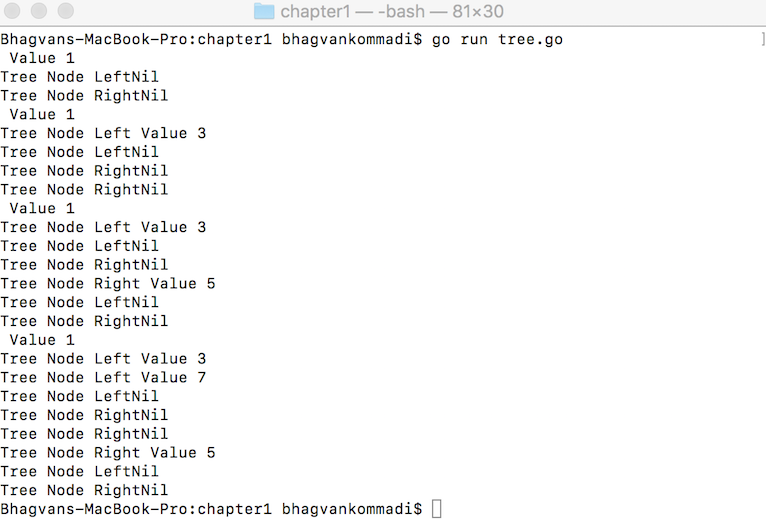Now that we know about the complexities in algorithms and analyzing their performance, let's take a look at brute force algorithms in the next section.

## Brute force algorithms

A brute force algorithm solves a problem based on the statement and the problem definition. Brute force algorithms for search and sort are sequential search and selection sort. Exhaustive search is another brute force algorithm where the solution is in a set of candidate solutions with definitive properties. The space in which the search happens is a state and combinatorial space, which consists of permutations, combinations, or subsets.

Brute Force algorithms are known for wide applicability and simplicity in solving complex problems. Searching, string matching, and matrix multiplication are some scenarios where they are used. Single computational tasks can be solved using brute force algorithms. They do not provide efficient algorithms. The algorithms are slow and non-performant. Representation of a brute force algorithm is shown in the following code:

```//main package has examples shown
//in Hands-On Data Structures and algorithms with Go book
package main
// importing fmt package
import (
"fmt"
)
//findElement method given array and k element
func findElement(arr int, k int) bool {
var i int
for i=0; i< 10; i++ {
if arr[i]==k {
return true
}
}
return false
}
// main method
func main() {
var arr = int{1,4,7,8,3,9,2,4,1,8}
var check bool = findElement(arr,10)
fmt.Println(check)
var check2 bool = findElement(arr,9)
fmt.Println(check2)
}```

Â

Â

Â

Â

Run the following commands:

`go run bruteforce.go`

The following screenshot displays the output: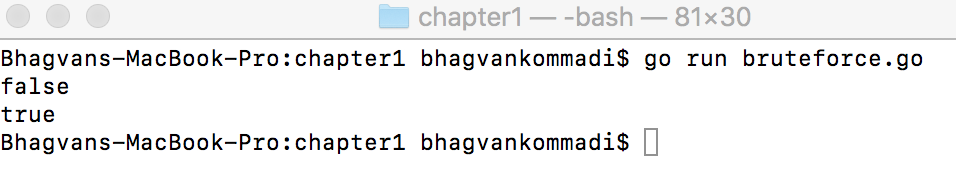After brute force algorithms, let's cover divide and conquer algorithms in the next section.

## Divide and conquer algorithms

A divide and conquer algorithm breaks a complex problem into smaller problems and solves these smaller problems. The smaller problem will be further broken down till it is a known problem. The approach is to recursively solve the sub-problems and merge the solutions of the sub-problems.

Recursion, quick sort, binary search, fast Fourier transform, and merge sort are good examples of divide and conquer algorithms. Memory is efficiently used with these algorithms. Performance is sometimes an issue in the case of recursion. On multiprocessor machines, these algorithms can be executed on different processors after breaking them down into sub-problems. A divide and conquer algorithm is shown in the followingÂ code:

```//main package has examples shown
// in Hands-On Data Structures and algorithms with Go book
package main
// importing fmt package
import (
"fmt"
)```

As shown in the following code, the Fibonacci method takes theÂ kÂ integer parameter and returns the Fibonacci number for k. The method uses recursion to calculate the Fibonacci numbers. The recursion algorithm is applied by dividing the problem into theÂ `k-1` integer and theÂ `k-2`Â integer:

```// fibonacci method given k integer
func fibonacci(k int) int {
if k<=1{
return 1
}
return fibonacci(k-1)+fibonacci(k-2)
}
// main method
func main() {
var m int = 5
for m=0; m < 8; m++ {
var fib = fibonacci(m)
fmt.Println(fib)
}
}```

Run the following commands:

`go run divide.go`

The following screenshot displays the output: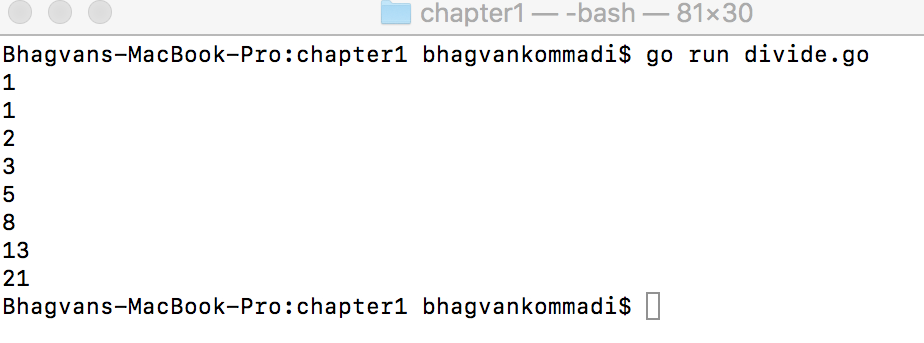Let's take a look at what backtracking algorithms are in the next section.

## Backtracking algorithms

A backtracking algorithm solves a problem by constructing the solution incrementally. Multiple options are evaluated, and the algorithm chooses to go to the next component of the solution through recursion. Backtracking can be a chronological type or can traverse the paths, depending on the problem that you are solving.

Backtracking is an algorithm that finds candidate solutions and rejects a candidate on the basis of its feasibility and validity. Backtracking is useful in scenarios such as finding a value in an unordered table. It is faster than a brute force algorithm, which rejects a large number of solutions in an iteration. Constraint satisfaction problems such as parsing, rules engine, knapsack problems, and combinatorial optimization are solved using backtracking.

The following is an example of a backtracking algorithm. The problem is to identify the combinations of elements in an array of 10 elements whose sum is equal to `18`. The `findElementsWithSum`Â method recursively tries to find the combination. Whenever the sum goes beyond the `k` target, it backtracks:

```//main package has examples shown
// in Hands-On Data Structures and algorithms with Go book
package main
// importing fmt package
import (
"fmt"
)
//findElementsWithSum of k from arr of size
func findElementsWithSum(arr int,combinations int,size int, k int, addValue int, l int, m int) int {
var num int = 0
return -1
}
num = num +1
var p int =0
for p=0; p < m; p++ {
fmt.Printf("%d,",arr[combinations[p]])
}
fmt.Println(" ")
}
var i int
for i=l; i< size; i++ {
//fmt.Println(" m", m)
combinations[m] = l
l = l+1
}
return num
}
// main method
func main() {
var arr = int{1,4,7,8,3,9,2,4,1,8}
var combinations int
//fmt.Println(check)//var check2 bool = findElement(arr,9)
//fmt.Println(check2)
}```

Run the following commands:

`go run backtracking.go`

The following screenshot displays the output: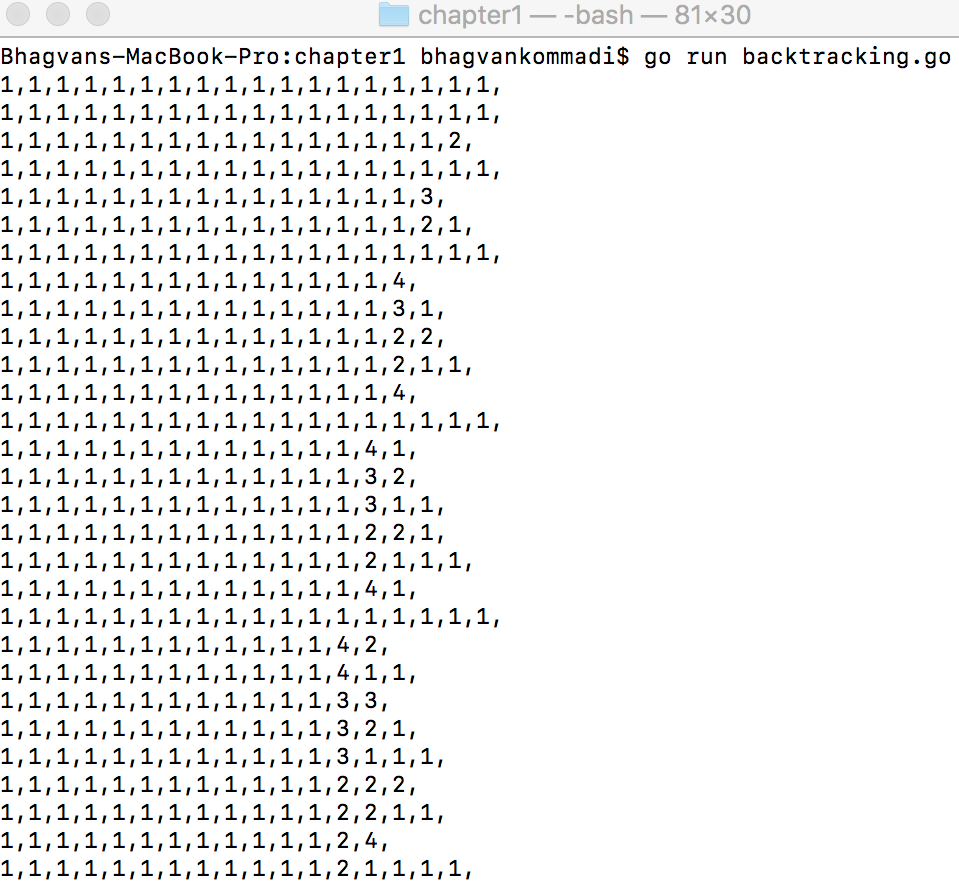Â

Â

## Summary

This chapter covered the definition of abstract datatypes, classifying data structures into linear, nonlinear, homogeneous, heterogeneous, and dynamic types. Abstract datatypes such as container, list, set, map, graph, stack, and queue were presented in this chapter. The chapter covered the performance analysis of data structures and structural design patterns.

We looked at the classification of data structures and structural design patterns. You can use algorithms such as brute force, divide and conquer, and backtracking by calculating the complexity and performance analysis. The choice of algorithm and the use of design patterns and data structures are the key takeaways.Â

In the next chapter, we will discuss data structures in Go. The following data structures will be covered:

• Arrays
• Slices
• Two-dimensional slicesÂ
• Maps

## Questions and exercises

1. Give an example where you can use a composite pattern.
2. For an array of 10 elements with a random set of integers, identify the maximum and minimum. Calculate the complexity of the algorithm.
3. To manage the state of an object, which structural pattern is relevant?
4. A window is sub-classed to add a scroll bar to make it a scrollable window. Which pattern is applied in this scenario?
5. Find the complexity of a binary tree search algorithm.
6. Identify the submatrices of 2x2 in a 3x3 matrix. What is the complexity of the algorithm that you have used?
7. Explain with a scenario the difference between brute force and backtracking algorithms.

Â

1. A rules engine uses backtracking to identify the rules affected by the change. Show an example where backtracking identifies the affected rules.
2. Draw a flow chart for the algorithm of the calculation of profit-loss given the cost price, selling price, and quantity.
3. Write the pseudo code for an algorithm that compares the strings and identifies the substring within a string.

The following books are recommended if you want to find out more about Gang of Four design patterns, algorithms, and data structures:

• Design Patterns,Â by Erich Gamma, Richard Helm, Ralph Johnson, and John Vlissides
• Introduction to AlgorithmsÂ â€“ Third Edition, by Thomas H. Cormen, Charles E. Leiserson, Ronald L. Rivest, and Clifford Stein
• Data structures and Algorithms: An Easy Introduction, byÂ Rudolph Russell